Function Repository Resource:

# SerializeWithDefinitions

Serialize an expression along with any needed definitions so that it can be used in another session

Contributed by: Richard Hennigan (Wolfram Research)
 ResourceFunction["SerializeWithDefinitions"][expr] serializes expr to a ByteArray along with its dependent definitions.

## Examples

### Basic Examples (2)

Serialize an expression with dependent definitions:

 In:=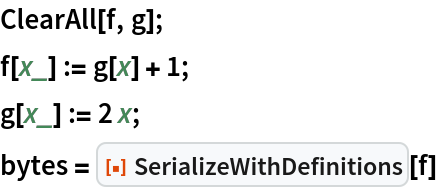Out=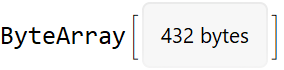Definitions are restored when the expression is deserialized:

 In:=Out=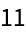### Generalizations and Extensions (2)

The serialized expression is an evaluation that restores definitions before returning the output:

 In:=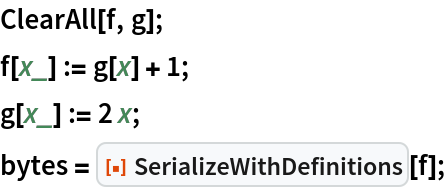In:=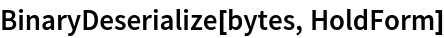Out=BinarySerialize by itself does not preserve definitions:

 In:=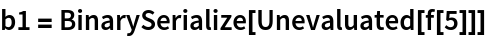Out=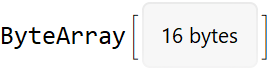In:=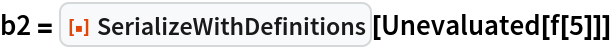Out=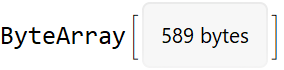In:=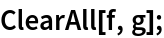In:=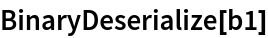Out=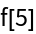In:=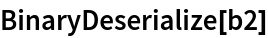Out=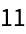### Options (3)

Serialize a Dataset:

 In:=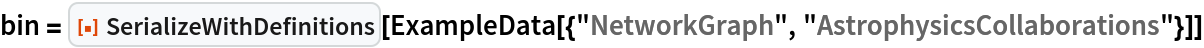Out=Serialize the same Dataset with PerformanceGoal set to "Size":

 In:=Out=Both forms represent the same expression:

 In:=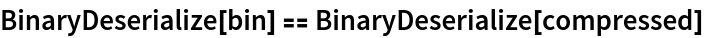Out=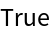### Applications (2)

CloudPut an evaluation that runs each time you use use CloudGet on it:

 In:=In:=Out=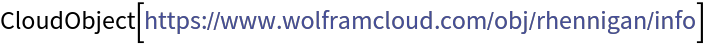In:=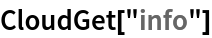Out=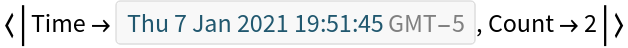In:=Out=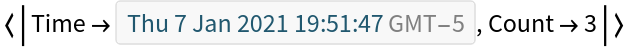Check the log:

 In:=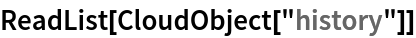Out=## Requirements

Wolfram Language 11.3 (March 2018) or above

## Version History

• 1.0.0 – 15 August 2018